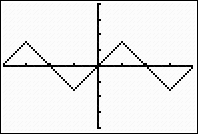Composition with Inverse Trig Functions

The graphs of the compositions of a trigonometric function with its inverse can yield some interesting results.  It is understood that a function and its inverse, when composed, return the original starting value -- they UNDO one another.  So, why does this "appear" to NOT be true when working with trigonometric functions on the graphing calculator?

Let's do some investigating: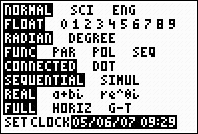The calculator is set to Radian mode.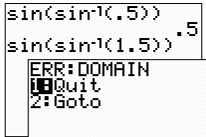The composition of a value within the limited domain of the sine inverse function, [-1,1], returns the starting value, just as expected.  If we, however, choose a value outside the domain of the sine inverse function, an error message is returned.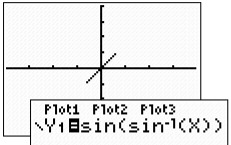The graph of the composition returns the identity line, y = x, for the domain of the sine inverse function from -1 to 1.  Our expected result of returning the starting value holds true as long as we remain within the domain of the sine inverse function.

Conclusion 1:  The calculator is seeing "sine inverse" to be ONLY the principal sine inverse function, and as such limits its domain to [-1,1].  All other domain values are seen as producing an error.  Consequently,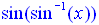, which starts with sin-1(x), is limited to accepting only values within the domain of  the "function" sin-1(x), which is [-1,1].  We see only these x-values being plotted which creates only a "segment" of the expected identity line y = x.

Let's REVERSE the order of the composition and see what happens:The calculator is set to Radian mode.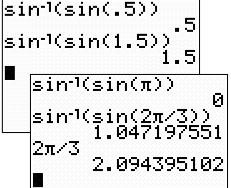No error message this time, but the "starting value" is not always being returned. The sin(x) function is now the starting function in the composition.  It has a domain of all Real values and always returns a result between -1 and 1 inclusive, which happens to be within the domain of the sine inverse function.  The sine inverse function will always return a value, but that value will be within its range of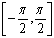.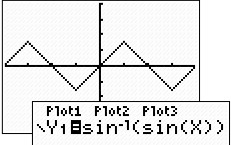Why are we seeing so much more? Since sin(x) is producing values in the range [-1,1], the sine inverse of these values can always yield a result and we see points plotted across the entire graph.  But this is not the expected y = x graph. The range of sine inverse isand it returns values only within this range.

Conclusion 2:  When the "starting" function in the composition is sine (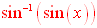), all numerical evaluations of the composition are possible, but the results will be restricted to the range of the inverse function.
When sin(x) produces positive values, the inverse function maps those values to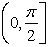in the first quadrant (or on the y-axis).   When sin(x) produces negative values, the inverse function maps those values to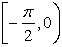in the fourth quadrant (or on the y-axis).

 When sin(x) = 0, sin-1(x) = 0. Consequently, the graph takes on the appearance of straight line segments alternating between positive and negative slopes, due to the restricted range of sine inverse to.